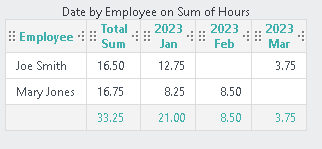•Here's an example of how you can achieve this.  Typically, the summary row and column formats are based on the crosstab value label's format.  If you used the wizard, most likely it applied the incorrect format to the label.

`<Report ID="LogiForum.CrosstabSummary">  <Body>    <CrosstabTable ID="CrosstabTable1" AjaxPaging="True" DraggableColumns="True" ResizableColumns="True" AccessibleSummary="AnalysisGrid CrossTabTable" Caption="Date by Employee on Sum of Hours" SortArrows="True">      <DataLayer Type="Static" ID="StaticDataLayer1">        <TimePeriodColumn ID="rdHdrGrpOp_Date_FirstDayOfMonth" DataColumn="Date" TimePeriod="FirstDayOfMonth" DateGroupByOperator="FirstDayOfMonth" DataType="Date" Format="yyyy MMM" />        <SortFilter SortColumn="rdHdrGrpOp_Date_FirstDayOfMonth" DataType="Date" />        <CrosstabFilter CrosstabColumn="rdHdrGrpOp_Date_FirstDayOfMonth" CrosstabLabelColumn="Employee" CrosstabValueColumn="Hours" CrosstabValueFunction="Sum">          <CrosstabRowSummaryColumn ID="AxSummaryRowValue" Function="Sum" />        </CrosstabFilter>        <StaticDataRow Date="3/10/2023" Employee="Joe Smith" Hours="3.75" />        <StaticDataRow Date="2/10/2023" Employee="Mary Jones" Hours="8.50" />        <StaticDataRow Date="1/8/2023" Employee="Joe Smith" Hours="3.25" />        <StaticDataRow Date="1/1/2023" Employee="Mary Jones" Hours="8.25" />        <StaticDataRow Date="1/1/2023" Employee="Joe Smith" Hours="9.5" />      </DataLayer>      <CrosstabTableLabelColumn ID="colAxLabel" Header="Employee">        <Label ID="lblAxLabl" Caption="@Data.Employee~" />        <DataColumnSort DataColumn="Employee" DataType="Text" />      </CrosstabTableLabelColumn>      <CrosstabTableLabelColumn ID="colAxSummary" Header="Total Sum" Note="This is for summary rows and columns.">        <DataColumnSort DataColumn="AxSummaryRowValue" DataType="Number" />        <Label ID="lblRowSummary" Caption="@Data.AxSummaryRowValue~" Format="###,###,##0.00" />        <DataColumnSummary ID="AxColumnSummary_CrosstabTable1" DataColumn="AxSummaryRowValue" Function="Sum" />      </CrosstabTableLabelColumn>      <CrosstabTableValueColumns ID="colAxValue" Header="@Data.rdCrosstabColumn~" Format="yyyy MMM">        <Label ID="lblAxValue" Caption="=iif(@Data.rdCrosstabValCount~=0,&quot;&quot;,&quot;@Data.rdCrosstabValue~&quot;)" Format="###,###,##0.00" />        <CrosstabValueColumnSort DataType="Number" />        <DataColumnSummary ID="AxSummaryValueSummary_CrosstabTable1" DataColumn="rdCrosstabColumn" Function="Sum" Format="#,###.#" DataType="Number" />      </CrosstabTableValueColumns>      <SummaryRow ID="AxSummaryHeaderRow" />      <InteractivePaging ShowPageNumber="True" PageRowCount="20" HideWhenOnePage="True" Location="Top" CaptionType="Image" rdIdeIdx="6" />    </CrosstabTable>  </Body>  <ideTestParams /></Report>`
••VISUI - many thanks for the prompt and detailed reply - we will take the information provided and see where it gets us!